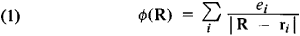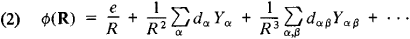# Multipole

Also found in: Dictionary, Medical, Wikipedia.

## multipole

[′məl·tə‚pōl]
(electromagnetism)
One of a series of types of static or oscillating distributions of charge or magnetization; namely, an electric multipole or a magnetic multipole.
McGraw-Hill Dictionary of Scientific & Technical Terms, 6E, Copyright © 2003 by The McGraw-Hill Companies, Inc.
The following article is from The Great Soviet Encyclopedia (1979). It might be outdated or ideologically biased.

## Multipole

a characteristic of a system of electric charges (“poles”) that has a definite symmetry. At a distance R from such a system (if R is sufficiently great compared to the system’s dimensions r), the static or variable electromagnetic field generated by the system can be visualized as a superposition of multipole fields of various orders / (a zero-order multipole is a charge, a first-order multipole is a dipole, a second-order multipole is a quadrupole, a third-order multipole is an octupole, and so on). For static fields the potential of an lth order multipole (the 2’-pole) decreases as 1/R l + 1 (if R « r) and also has a specific angular dependence. At distances R that are much greater than the wavelength of the emitted waves (in the wave zone), the variable (radiated) fields of oscillating multipoles of any order have an identical dependence on R (they vary as 1/ R) and can be distinguished only by their angular dependence, which is the same as that of static multipoles.

The magnitude and angular distribution of a multipole field, as well as the energy of its interaction with an external field, are determined by the multipole moment. If all multipole moments up to the order I —1 are equal to zero, the moment of the order L is independent of the selection of the coordinate origin within the system.

In the case of a static system of charges ei, located at points ri (with coordinates xia, where ɑ = 1, 2, 3), the potential ɸ(R) of a constant electric field at point R isFor large R (Rri), the potential may be represented as a series in powers of ri/Rwhere the scalar e = Σiei is the total charge of the system, the vector da = Σiexia is its dipole moment, and the tensor dαβ = Σiei (3xαβxαβ - ri2δαβ) is the quadrupole moment (where α, β = 1, 2, 3 and δαβ is the Kronecker symbol, which is equal to 1 for α = β and 0 for α ɸ β); the quantities Ya, Yαβ, and so on depend only on the direction of the vector R and are expressed in terms of spherical harmonics of the appropriate order I. In the simplest case a dipole field is generated by two charges of equal magnitude but opposite sign; a quadrupole field is generated by four charges of equal magnitude placed at the vertexes of a parallelogram such that each side connects charges of opposite sign; an octupole field is generated by eight charges in the vertexes of a parallelepiped, with each edge connecting opposite charges; and so on.

Magnetic multipoles are used to describe the magnetic properties of a system. Since magnetic charges do not exist, the resolution of vector potential, which is analogous to the resolution of the scalar potential Ф, starts with the magnetic dipole.

The resolution of a variable field by multipoles plays an important role in classical radiation theory and antenna theory. This approach is particularly useful in the quantization of the radiation field. The wave function of a radiation field of the 2’-pole is an eigenfunction of the operator of an angular momentum, with an eigenvalue of l: such a multipole radiates photons only with moment l.

The concept of a multipole is also used in describing variable acoustic, gravitational, and other fields.

### REFERENCES

Landau, L. D., and E. M. Lifshits. Teoriia polia, 6th ed. Moscow, 1973. (Teoreticheskaia fizika, vol. 2.)
Frenkel’, la. I. Elektrodinamika: Sobr. izbr. trudov, vol. 1. Moscow-Leningrad, 1956.
Akhiezer, A. I., and V. B. Berestetskii. Kvantovaia elektrodinamika, 3rd ed. Moscow, 1969.

V. P. PAVLOV

References in periodicals archive ?
Jiang, "The error control of mixed-form fast multipole algorithm based on the high-order multipole rotation," IEEE Antenn.
Zhao, "Acceleration of fast multipole method for large-scale periodic structures with finite sizes using sub-entire-domain basis functions," IEEE Transactions on Antennas and Propagation, vol.
Chang, "A line integration method for the treatment of 3D domain integrals and accelerated by the fast multipole method in the BEM," Computational Mechanics, vol.
[mathematical expression not reproducible] are called multipole moments presented by Wang and Yao ; [S.sub.n,m] is called solid spherical harmonic function .
PITSIANIS, A matrix version of the fast multipole method, SIAM Rev., 43 (2001), pp.
Another more obvious example illustrates the SCD when using multipole relays: Two matrices are interconnected on their Y1 and Y2 lines via a two-pole relay (Figure 5).
You could be a very good analyst, but you were overwhelmed by multipole expansion.
"Multipole analysis of photonic crystal fibers with coated inclusions".
Dispersion parameters as well as the modal field were calculated by using CUDOS MOF Utilities  that simulate PCF with multipole method [22, 23].
Ohkawa work for Kerst doing experiments on "multipole" configurations and soon became recognized for his intellect and innovative contributions.
Two methods are presented to assess the GHE performances by drawing a box plot and a surface chart of the borehole thermal resistance using the Remund (1999) and multipole (Claesson and Hellstrom 2011) methods, respectively, and carrying out a comparison with the effective borehole thermal resistance inferred in the field.

Site: Follow: Share:
Open / Close